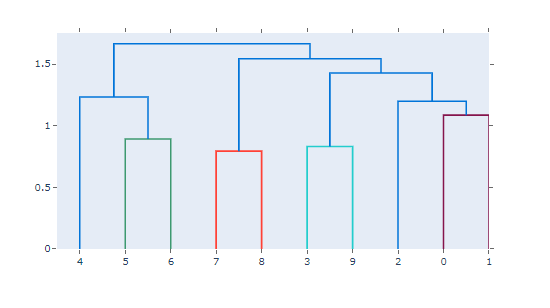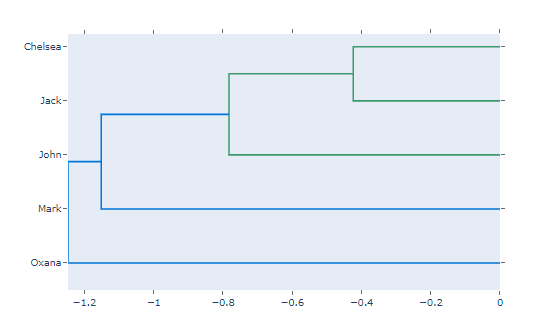Skip to content
Related Articles
plotly.figure_factory.create_dendrogram() function in Python
• Last Updated : 26 Jul, 2020

Plotly library of Python can be very useful for data visualization and understanding the data simply and easily.

## plotly.figure_factory.create_dendrogram

A dendrogram is a diagram representing a tree. The figure factory called create_dendrogram performs hierarchical clustering on data and represents the resulting tree. Values on the tree depth axis correspond to distances between clusters.

Syntax: plotly.figure_factory.create_dendrogram(X, orientation=’bottom’, labels=None, colorscale=None, distfun=None, linkagefun=<function <lambda>>, hovertext=None, color_threshold=None)

Parameter:

X ((ndarray)) – it describes the matrix of observations as array of arrays

orientation ((str)) – in this we use ‘top’, ‘right’, ‘bottom’, or ‘left’

labels ((list)) – it describes the list of axis category labels(observation labels)

colorscale ((list)) – it describes the optional colorscale for dendrogram tree

distfun ((function)) – it describes the function to compute the pairwise distance from the observations

linkagefun ((function)) – it describes the function to compute the linkage matrix from the pairwise distances

hovertext ((list[list])) – it describes the list of hovertext for constituent traces of dendrogram clusters

color_threshold ((double)) – it describes the value at which the separation of clusters will be made

Example 1: Simple bottom oriented dendrogram

## Python3

 `from` `plotly.figure_factory ``import` `create_dendrogram``import` `numpy as np`` ` ` ` `X ``=` `np.random.rand(``10``,``10``)``fig ``=` `create_dendrogram(X)``fig.show()`

Output:Example 2:  Dendrogram to put on the left of the heatmap

## Python3

 `from` `plotly.figure_factory ``import` `create_dendrogram``import` `numpy as np`` ` ` ` `X ``=` `np.random.rand(``5``,``5``)``names ``=` `[``'Jack'``, ``'Oxana'``, ``'John'``, ``'Chelsea'``, ``'Mark'``]`` ` `dendro ``=` `create_dendrogram(X, orientation``=``'right'``, labels``=``names)``dendro.update_layout({``'width'``:``700``, ``'height'``:``500``}) ``dendro.show()`

Output:Attention geek! Strengthen your foundations with the Python Programming Foundation Course and learn the basics.

To begin with, your interview preparations Enhance your Data Structures concepts with the Python DS Course. And to begin with your Machine Learning Journey, join the Machine Learning – Basic Level Course

My Personal Notes arrow_drop_up